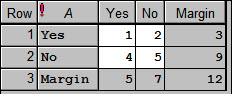1. Home
2. Table Calculation Options

# Table Calculation Options

Controls the options used when using tables in calculations.## Summary of results

Displays a summary of the results of the calculation.

## Zero divided by zero

The quantity 0/0 is not defined mathematically. In Genstat calculations the result of 0/0 can be stored as 0 or missing value (*).

## Include table margins using

When selected, margins will be added to the resulting table using the statistic selected in the dropdown list. For example, in an A x B table if the totals are used to calculate the margin then A’s margin will be the total/sum of the B classifying factor cells for each level of A, and vice-versa for B. The image below shows a table with margins formed using totals.